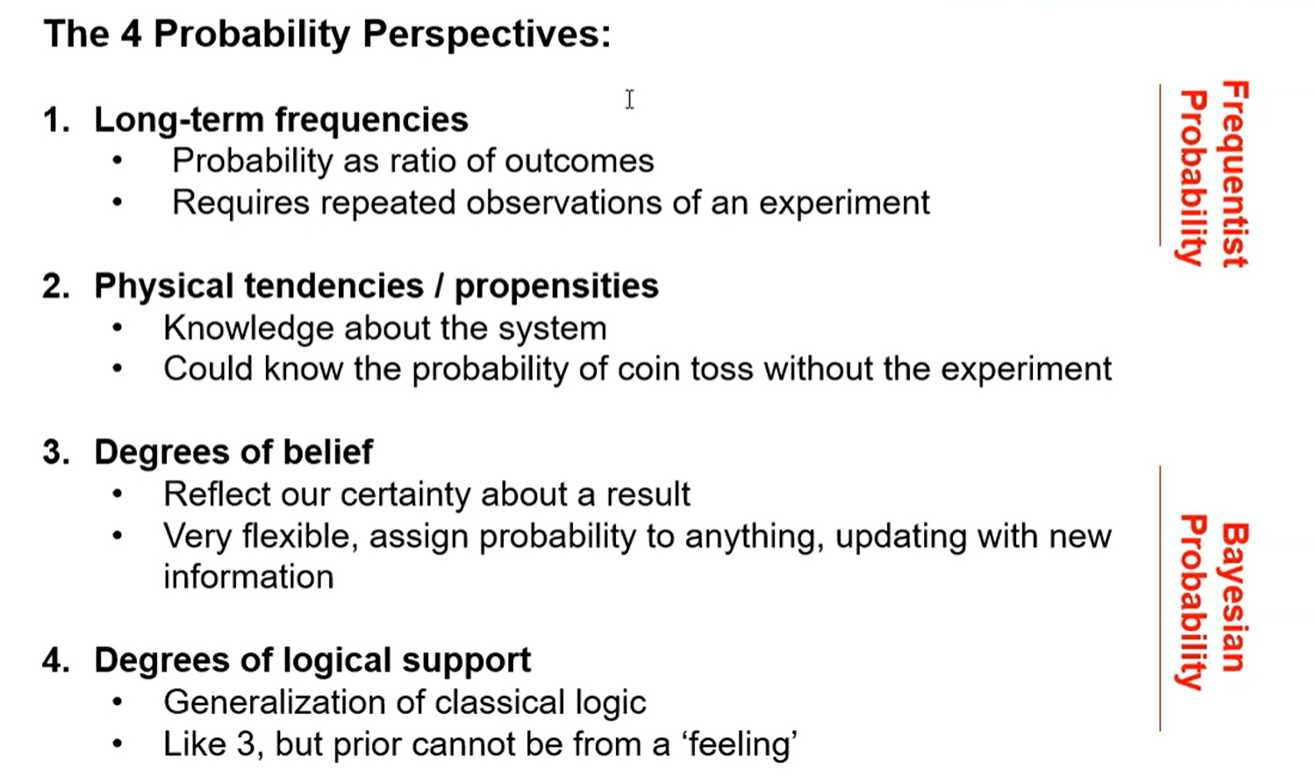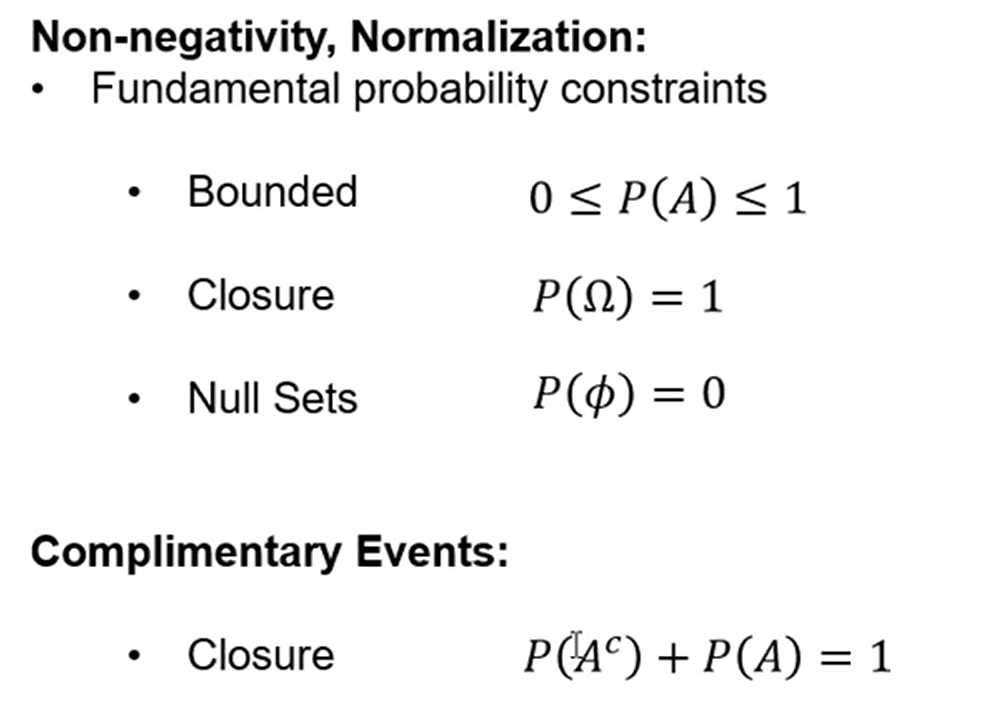# Sampling representatively

Random Sampling: when every item in the population has an equal chance of being chosen. Selection of every item is independent of every other selection. Is random sampling sufficient for subsurface? Is it available?
- it is not usually available, would not be economic
- data is collected answer questions
- how large is the reservoir, what is the thickest part of the reservoir
- and wells are located to maximize future production
- dual purpose appraisal and injection / production wells
Regular Sampling: when samples are taken at regular intervals (equally spaced).
- Less reliable than random sampling.
- Warning: May resonate with some unsuspected environmental variable.
What do we have?
- we usually have biased, opportunity sampling
- we must account for bias (debiasing will be discussed later)

# Data collection

If we were sampling for representativity of the sample set and resulting sample statistics, by theory we have 2 options:
1. random sampling
2. regular sampling (as long as we don’t align with natural periodicity)
What would happen if you proposed random sampling in the Gulf of Mexico at \$150M per well?

We should not change current sampling methods as they result in best economics, we should address sampling bias in the data.

Never use raw spatial data without access sampling bias
correcting.

## There are also limits to our data collection:

• accessibility to the sample — obstruction, reliable drilling, subsalt imaging
• inability to process the sample — may not be able to recover shale core Lsamples
• can’t run permeability evaluation on low permeability rock

# Sampling definitions

• Variable: any property measured / observed in a study
• e.g. porosity, permeability, mineral concentrations, saturations, contaminant concentration
• in data mining / machine learning this is known as a feature
• Population: Exhaustive, finite lisi of property of interest over area of interest. Generally the entire population is not accessible.
• e.g. porosity at each location within a reservoir.
• Sample: The set of data that have actually been measured
• e.g. porosity data from measured by well-logs within a reservoir.
• Parameters: summary measure of a population
• e.g. population mean, population standard deviation, we rarely have access to this.
• Statistics: summary measure of a sample
• e.g. sample mean, sample standard deviation, we use statistics as estimates of the parameters.
• Sampling Bias: we must assume all of our sparsely sampled data is sampled in a bias manner, more on debiasing later!# What is probability

A Measure that Honors Kolmogorov's 3 Axioms:
1. Probability of an event is a non-negative number.

\operatorname{Prob}(A) \geq 0

1. Unit Measure, probability of the entire sample space is one (unity).

\operatorname{Prob}(\Omega)=1

1. Additivity of mutually exclusive events for unions.

\operatorname{Prob}\left(\bigcup_{i=1}^{\infty} A_{i}\right)=\sum_{i=1}^{\infty} \operatorname{Prob}\left(A_{i}\right)

e.g. probability of A_{1} and A_{2} mutual exclusive events is \operatorname{Prob}\left(A_{1}\right)+\operatorname{Prob}\left(A_{2}\right)